• 2020-12-08 07:16:38

您可以使用np.einsum进行操作,因为它可以非常小心地控制哪些轴相乘,哪些相加：

>>> np.einsum('ijk,ij->ik', ind, dist)

array([[ 0.4, 0.4, 0.4, 0.4],

[ 3. , 3. , 3. , 3. ],

[ 1. , 1. , 1. , 1. ]])

该函数将ind的第一个轴中的条目与dist(下标’i’)的第一个轴中的条目相乘.同一个数组的第二个轴(下标“j”).而不是返回一个3D数组,我们告诉einsum通过从输出下标中省略它来沿轴’j’求和,从而返回一个2D数组.

np.tensordot更难适用于这个问题.它自动求和轴的乘积.但是,我们想要两套产品,但只要求其中之一.

写入np.tensordot(ind,dist,axes = [1,1])(如您所链接的答案)为您计算正确的值,但返回一个具有形状(3,4,3)的3D数组.如果您能负担更大阵列的内存成本,您可以使用：

np.tensordot(ind, dist, axes=[1, 1]).T

这给了你正确的结果,但是因为tensordot首先创建一个大于必要的阵列,所以einsum似乎是一个更好的选择.

更多相关内容
• I have two matrix:A = [a11 a12a21 a22]B = [b11 b12b21 b22]And I want to multiply all its columns (without loops) in order to obtain the matrix:C =[a11*b11 a11*b12 a12*b11 a12*b12a21*b21 a21*b2...I have two matrix:

A = [a11 a12

a21 a22]

B = [b11 b12

b21 b22]

And I want to multiply all its columns (without loops) in order to obtain the matrix:

C =[a11*b11 a11*b12 a12*b11 a12*b12

a21*b21 a21*b22 a22*b21 a22*b22]

I've tried with

>>> C = np.prod(A,B,axis=0)

but prod doesn't accept two input matrix. Neither np.matrix.prod.

解决方案

We could use broadcasting for a vectorized solution -

(A[...,None]*B[:,None]).reshape(A.shape,-1)

Philosophy : In terms of vectorized/broadcasting language, I would describe this as spreading or putting the second dimension of the input arrays against each other, while keeping their first dimensions aligned. This spreading is done by introducing new axes with None/np.newaxis for these two inputs and then simply multiplying each other.

Mathematical view : Let's use a bit more mathematical view of it with the help of a generic example. Consider input arrays having different number of columns -

In : A = np.random.rand(2,3)

In : B = np.random.rand(2,4)

First off, extend the dimensions and check their shapes -

In : A[...,None].shape

Out: (2, 3, 1)

In : B[:,None].shape

Out: (2, 1, 4)

Now, perform the element-wise multiplication, which will perform these multiplications in a broadcasted manner. Take a closer look at the output's shape -

In : (A[...,None]*B[:,None]).shape

Out: (2, 3, 4)

So, the singleton dimensions (dimension with length = 1) introduced by the use of None/np.newaxis would be the ones along which elements of the respective arrays would be broadcasted under the hood before being multiplied. This under-the-hood broadcasting paired with the respective operation (multiplication in this case) is done in a very efficient manner.

Finally, we reshape this 3D array to 2D keeping the number of rows same as that of the original inputs.

Sample run :

In : A

Out:

array([[2, 3],

[4, 5]])

In : B

Out:

array([[12, 13],

[14, 15]])

In : (A[...,None]*B[:,None]).reshape(A.shape,-1)

Out:

array([[24, 26, 36, 39],

[56, 60, 70, 75]])

NumPy matrix type as inputs

For NumPy matrix types as the inputs, we could use np.asmatrix that would simply create view into the inputs. Using those views, the broadcasted element-wise multiplication would be performed, finally resulting in a 2D array after the reshaping. So, the last step would be to convert back to np.matrix type. Let's use the same sample inputs to demonstrate the implementation -

In : A

Out:

matrix([[2, 3],

[4, 5]])

In : B

Out:

matrix([[12, 13],

[14, 15]])

In : arrA = np.asarray(A)

In : arrB = np.asarray(B)

In : np.asmatrix((arrA[...,None]*arrB[:,None]).reshape(A.shape,-1))

Out:

matrix([[24, 26, 36, 39],

[56, 60, 70, 75]])

展开全文• 什么是向量 向量是空间中有方向的线段 简单的理解可以认为向量是一个箭头，比如我要导航去一个地方，导航告诉我先向前走多少多少米，然后右转多少多少米。其实它是两个带有方向的线段，直走了一段，又右拐走了一段。...

参考学习教程：慕课网《大学计算机必修新讲》
文章图片来源：慕课网《大学计算机必修新讲》

# 什么是向量

向量是空间中有方向的线段
简单的理解可以认为向量是一个箭头，比如我要导航去一个地方，导航告诉我先向前走多少多少米，然后右转多少多少米。其实它是两个带有方向的线段，直走了一段，又右拐走了一段。

一组数据
还有一种向量他就是一组数据。其实它也是有方向的，但方向已经没有那么明显了。比如我的猫猫身长+体重的数据（17.5cm，3.5kg）。这一组数据也称为向量，它通常是做数据，做AI。

# 二维平面的向量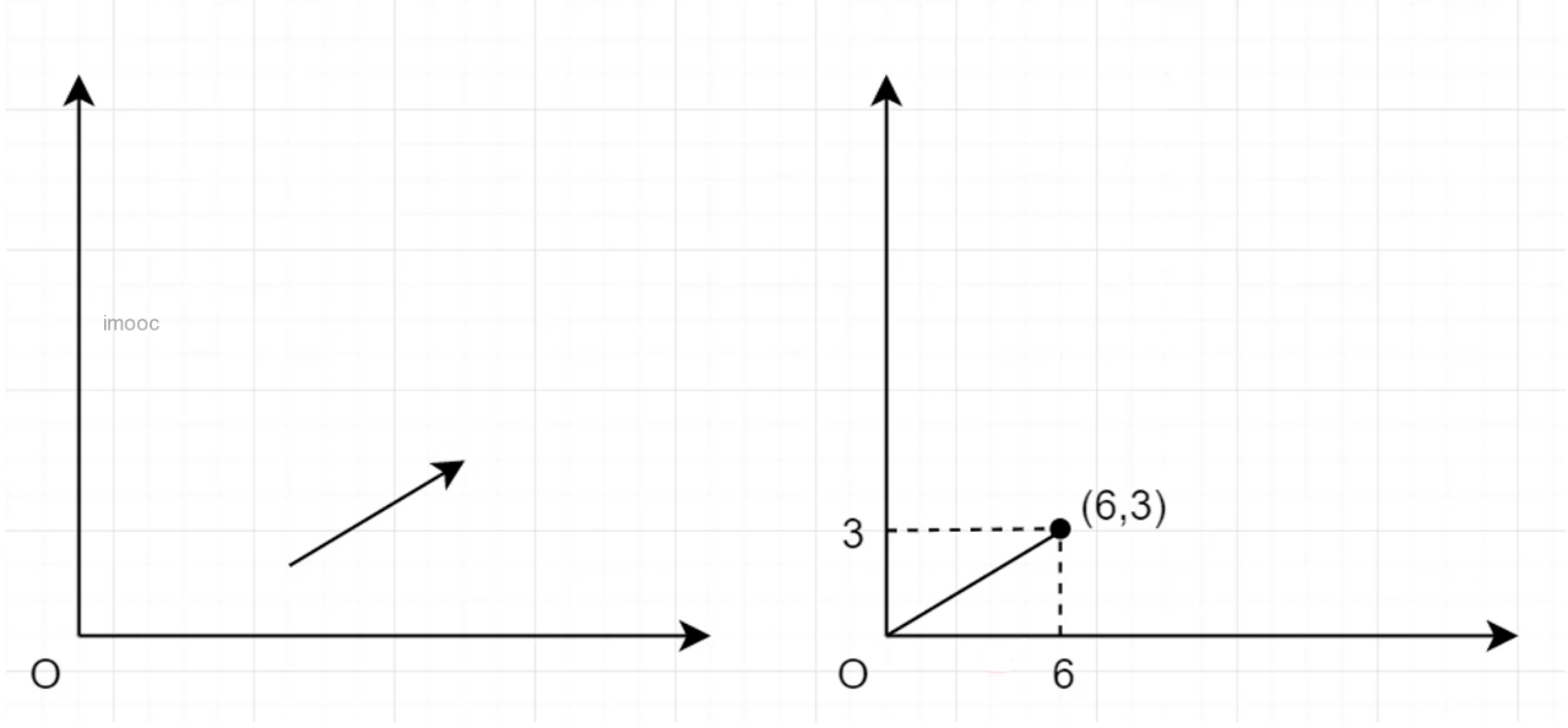如上图所示，左边的箭头和右边是一样长的，箭头不管移动到哪里都是一样的。向量不在乎在哪里，它只在乎方向和箭头。可以以（0，0）开始，可以给向量一个坐标。在一个二维平面可以用坐标来表示向量。

# 三维直角坐标系

如果放到一个直角坐标系（也叫做笛卡尔坐标系），向量的坐标会变成三个值。原本的二维坐标系，x,y轴，再增加一个垂直的z轴。它的轴之间是互相垂直的关系。
x轴和y轴确定之后，z轴有两个方向，如下图，z轴可以向里或者向外。就有了左手系和右手系的区别。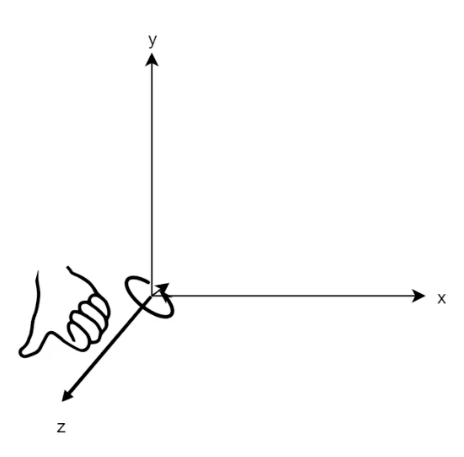想象自己的右手握住z轴，用大拇指指向z轴的方向。 观察自己手指的方向握住的时候是从x轴往y轴转的时候就是右手系，相反y轴转向x轴就是左手系。所以右手系到左手系的转变很简单，把z从正值变成负值。
右手系很适合人的思维，如图画x，y，z轴向外画会增加表现力。但是真正图形渲染引擎在绘制系统的时候是用左手系。xy轴就可以看作是屏幕，z轴向越向里面，就会越小。

# 三维的空间向量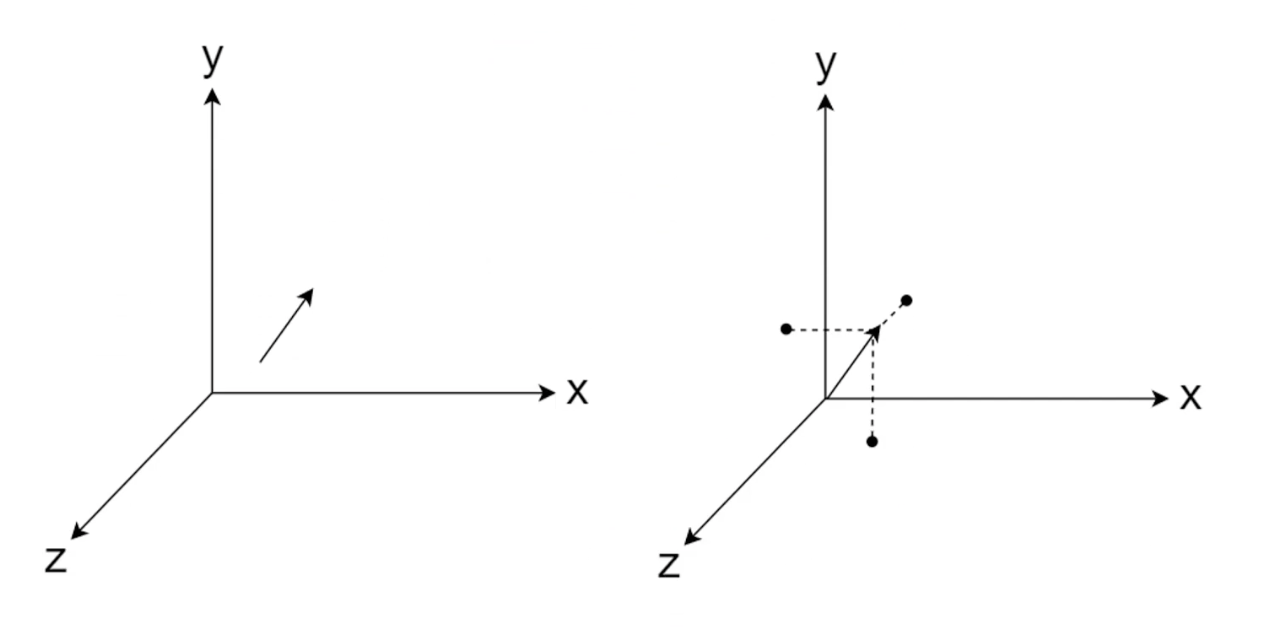三维空间的向量同理二维坐标系，把左侧的向量移到远点，取向量的x,y,z。
先做一个投影到（x,z）平面，然后画一条垂直的直线到x轴找到x的值，y值就是投影到（x,y）平面，然后画平先行到y轴。其实也取x值也可以投影到(x,y)平面取垂直的到轴。
最终向量如果把起点放到圆点，这时会有一个延伸出去的线段，线段端点的坐标就是这个向量。

# 向量的表示

向量的表示会有三种方法：

1. 用括号表示
2. 用矩阵的方式，向量经常和矩阵在一起做运算。矩阵的运算就是一些线性变化，平移，旋转，缩放
3. 用单位向量几何的表示，用箭头代表是一个向量。如下图二维表示就是3倍的i向量+1倍的j向量代表最终的距离。

二维向量表示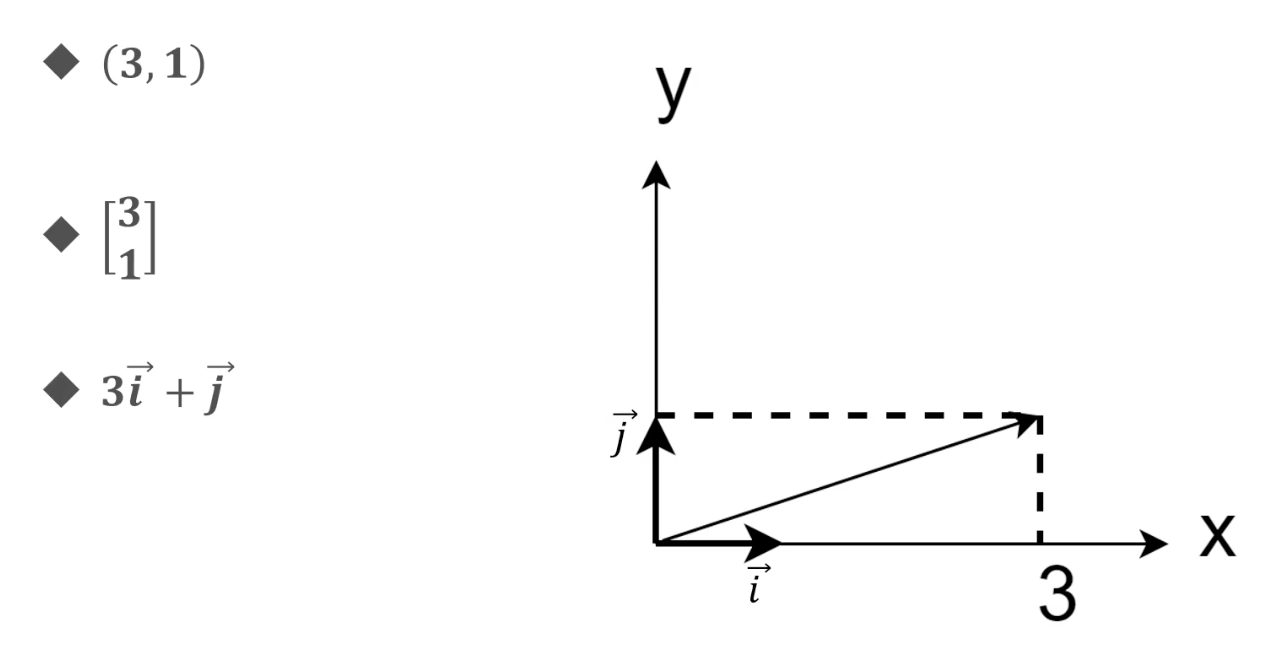三维向量表示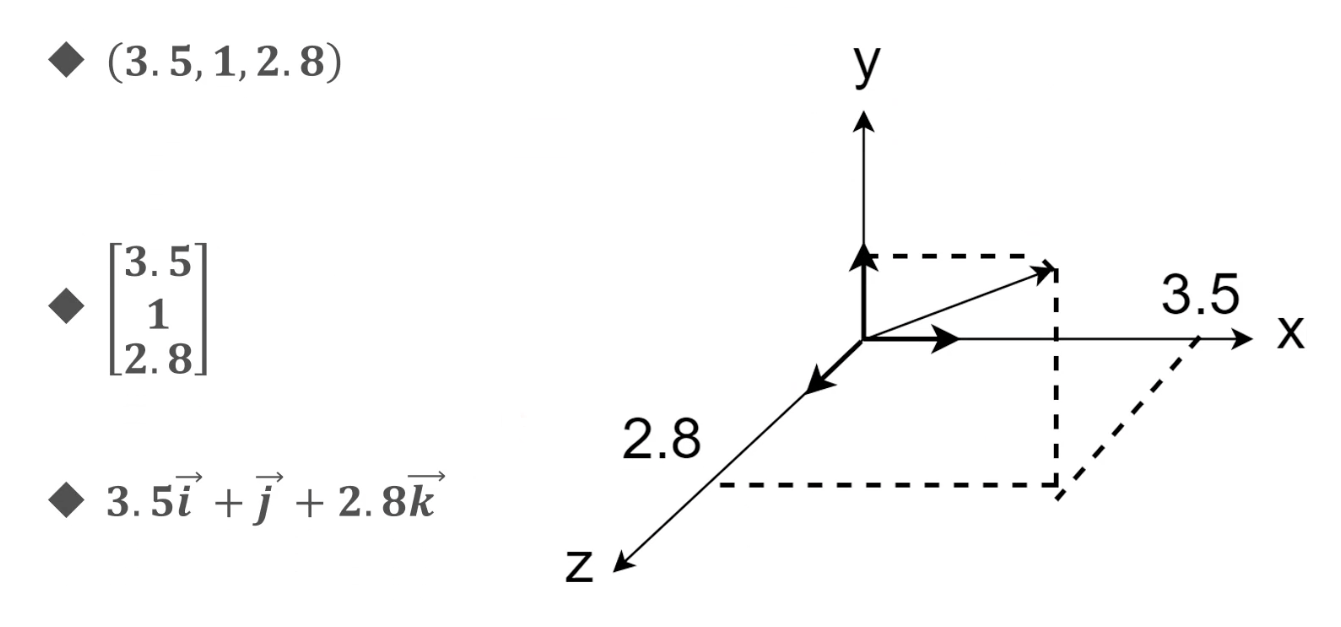向量始终遵循勾股定理。

# 向量和是什么

向量代表地图上的方向。如下图，最终向量AB+向量BC+向量CD就是向量。A-D的向量就是紫色的线段。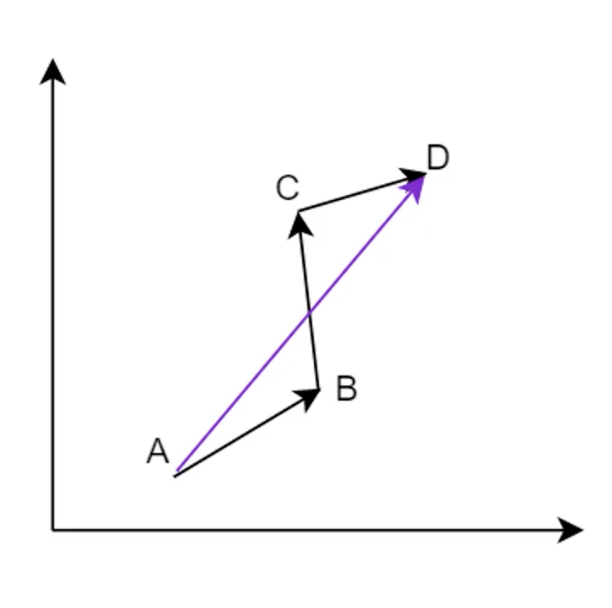# 向量和

比如（1，2）+（2，3） = （3，5）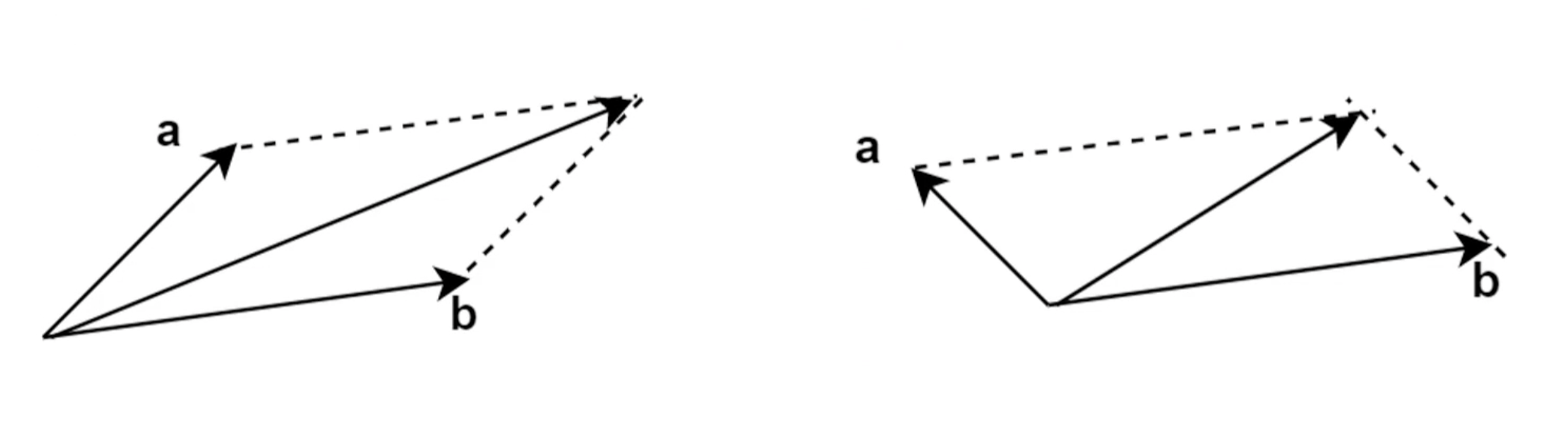可以把向量和理解成沿着a走，再沿着b走。从初始位置到终点是平行四边形的对角线。

# 向量乘以常数

向量的乘法可以看作多次加法。
假设向量(1,2,3)*3可以看作是 (1,2,3)+(1,2,3)+(1,2,3) = (3,6,9)
向量乘以常数可以看作向量成倍的延长，负数倍数会反过来。

# 向量的长度

向量的长记做|A|（A上面有一个向右的箭头，我打不出来），读做向量的模。
长度遵循勾股定理，A（x,y）他就等于x2+y2平方开根。
三维向量长度也是如此。A(x,y,z)。向量长度等于x2+y2+z2开平方根

# 线性组合

给定两个向量Va和Vb，还有两个常数x，y
Vc = xVa+yVb，如下图，用a和b组合成向量c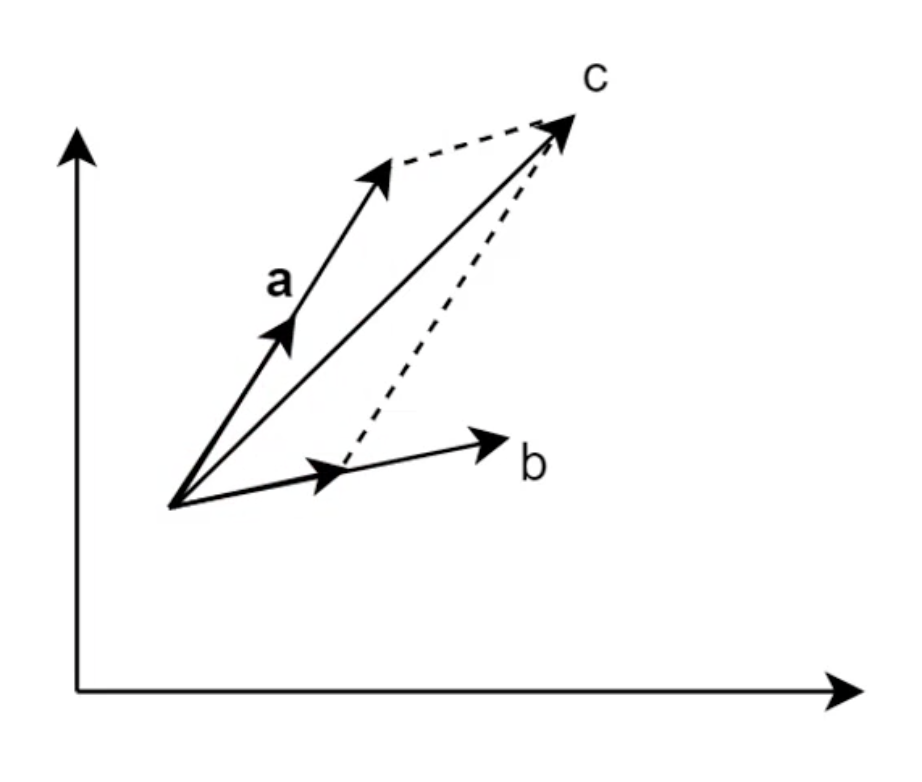也可以组合如下：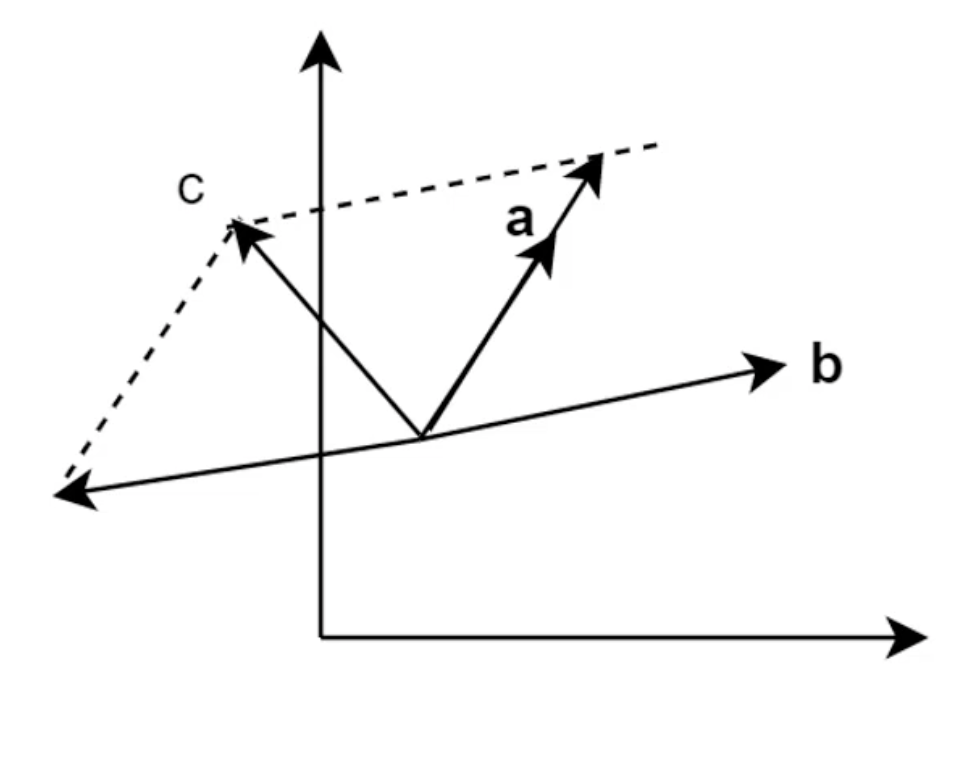只要向量a和向量c满足一定条件（两条向量不能平行）就可以代表任何向量。同理，在三维空间也适用。如下图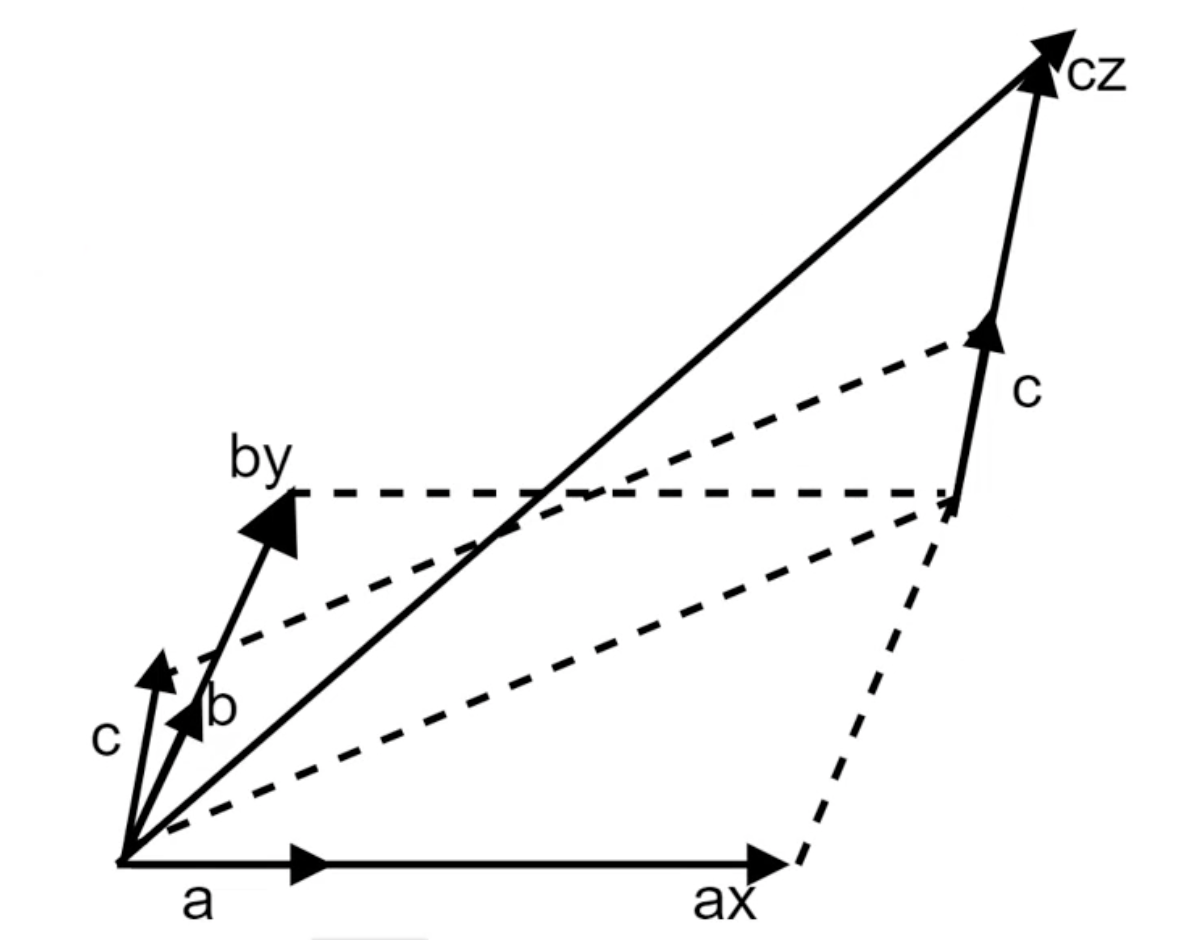向量a和向量b延长得到的向量，在和向量c的延长就可以得倒新的向量，相当于做了两个平行四边形，取第二次平行四边形的对角线。

展开全文• 我需要编写一个程序来自行乘以矩阵(nXn)。我必须计算过程中的最大值和最小值，而不是最后。这是我的代码：import csvimport timestart= time.time()matrix = []with open('matrix.csv', newline='') as csvfile:exc1...

我需要编写一个程序来自行乘以矩阵(nXn)。

我必须计算过程中的最大值和最小值，而不是最后。

这是我的代码：

import csv

import time

start= time.time()

matrix = []

with open('matrix.csv', newline='') as csvfile:

for row in exc1:

matrix.append(row)

matrix_length=len(matrix)

min = 9999999999999999999999999999999999999999999

max = 0

result = [[None]*matrix_length for _ in range(matrix_length)]

for i in range(matrix_length):

for j in range(matrix_length):

for k in range(0,matrix_length):

result[i][j] += int(matrix[i][k]) * int(matrix[k][j])

if (int(result[i][j])

min = int(result[i][j])

if (int(result[i][j])>max):

max = int(result[i][j])

done=time.time()

print("max:",max)

print("min:",min)

print(done-start)这是错误：

File "", line 24, in

result[i][j] += int(matrix[i][k]) * int(matrix[k][j])

TypeError: unsupported operand type(s) for +=: 'NoneType' and 'int'你明白什么是问题吗？

展开全文• 矩阵部分运算代码 ...%矩阵乘以常数 AA=A*(0.017576/729000); M=[0.4056 0 0; 0 0.4056 0 0 0 0.4056]; %两个矩阵相乘 D = AA * M; %求矩阵D的特征向量，存在d里;特征值，存在v里 [d,v] = eig(D) ...matlab
• 1、Matlab向量的生成方法(1)直接输入法(2)冒号生成法(3)线性等分法2、直接输入法>> a=[1,2,3,4;5,6,7,8;9,10,11,12]a =1 2 3 45 6 7 89 10 11 123、冒号生成法>> b=1:2:100b =Columns 1 through 171 3.....
• 关于向量 高中数学必修444说: 几何向量是线性空间中有大小与方向的量。 放图理解一下： 如上图所示，向量可以形象的用一根箭头表示。箭头所指代表向量的方向，线段的长度代表向量的大小。 在OIOIOI中，...
• 向量和标量的计算，babylon.js和three,js的区别。 代码： //three.js var THREE = require('./js/libs/three.js') //babylon.js var BABYLON = require('./js/libs/babylon.js') //threeVector var ...
• 特征向量确实有很明确的几何意义，矩阵(既然讨论特征向量的问题，当然是方阵，这里不讨论广义特征向量的概念，就是一般的特征向量)乘以一个向量的结果仍是同维数的一个向量，因此，矩阵乘法对应了一个变换，把一个...存储 算法 matlab 图像处理
• 线性代数向量乘法Prerequisite: Linear Algebra | Defining a Vector 先决条件： 线性代数| 定义向量 Linear algebra is the branch of mathematics concerning linear equations by using vector spaces and ...python 机器学习 神经网络 算法
• 矩阵与常数的运算MATLAB 在线性代数中的应用— 在矩阵的运算上的应用 2．矩阵与常数的运算 (1)数加 矩阵与常数的加指矩阵的每个元素都加 上此常数。 (2)数乘 矩阵与常数的乘指矩阵的每个元素都乘 上此常数。 (3)数除...
• 向量的点乘、叉乘等操作。 代码 double ThreeDot(Point p,Point a,Point b){ return(a-p)*(b-p)+(a-p)*(b-p)+(a-p)*(b-p); }//pa与pb的点积 (3点) Point ThreeCross(Point...点乘 c++ 代码
• 我们可以把原坐标系的(x,y)乘以一个矩阵，得到一个新的(x’,y’)的表示形式，写为算子的形式就是(x,y)M=(x’,y’)。这里的矩阵M代表一种线性变换：拉伸，平移，旋转。那么，有没有什么样的线性变换b(b是一
• 当孔乙己说回字有四样写法的时候，相信各位都是这样的表情吧？...为了在写作和阅读时保持清晰的逻辑和清醒的头脑，本文仅对四种最常见的数组乘法给出详细说明，并用一道数学题来演示向量点乘和叉乘的用法。
• 特征向量确实有很明确的几何意义，矩阵(既然讨论特征向量的问题，当然是方阵，这里不讨论广义特征向量的概念，就是一般的特征向量)乘以一个向量的结果仍是同维数的一个向量，因此，矩阵乘法对应了一个变换，把一个...
• 在学习吴恩达教授机器学习时，其中讲解到关于矩阵向量部分。由此记录。 概念 矩阵实例 下面的矩阵用A来代替 向量 向量和矩阵相乘时，用矩阵的每一行去和向量的每一列相乘 即得到的第一个值为 a1 * b1+a2 * b2. ...c# 机器学习
• 1、矩阵*乘列向量 a=np.array([[1,2],[3,4]]) a array([[1, 2], [3, 4]]) b=np.array([,]) b array([, ]) a*b ans=a*b ans array([[ 2, 4], [ 9, 12]]) 2、矩阵*乘常数 ans=a*2 ans array([[2, 4
• 线性代数向量乘法Prerequisites: 先决条件： Defining a Vectors 定义向量 Linear algebra is the branch of mathematics concerning linear equations by using vector spaces and through matrices. In other ...python 机器学习 算法 神经网络
• 指定一个注释并指定格式 _ 引用符号和转置运算符 ._ 非共轭转置运算符 = 赋值运算符 特殊变量和常数 名称 含义 ans 最近的回应/回答 eps 浮点精度精度 pi 数字π Inf ∞ i, j 虚构单位√-1 .Nan 未定义的数值结果,...
• //矩阵和向量的乘积 int F[N][R],H[M][R]; printf(“请输入矩阵F(34):\n"); for(i=0;i for(j=0;j scanf("%d",&F[i][j]); } } for(i=0;i for(j=0;j H[i][j]=0; } } printf("矩阵H（AF）为:\n”); for(i=0;i for(j=0;...
• 平面向量的数量积平面向量数量积的定义已知两个非零向量a和b，它们的夹角为θ，把数量|a||b|cosθ叫做a和b的数量积(或内积)，记作a·b.即a&m...积化和差，指初等数学三角函数部分的一组恒等式。可以通过展开角的...
• 两个向量的叉积是这样表示的： ，这种乘法的计算结果是另一个矢量 ，这个矢量 的大小等于原来两个矢量的大小的乘积再乘以两个矢量夹角 (小于180度)的「正弦」： ，在二维空间内，向量其几何意义就是以两个向量为边的...叉乘 线性代数
• 矩阵A乘以x表示，对向量x进行一次转换（旋转或拉伸）（是一种线性转换），而该转换的效果为常数c乘以向量x（即只进行拉伸）。 我们通常求特征值和特征向量即为求出该矩阵能使哪些向量（当然是特征向量）只发生拉伸，...
• 向量（Vector3） 在虚拟的游戏世界中，3D数学决定了游戏，如何计算和模拟出开发者以及玩家看到的每一帧画面。学习基础的3D数学知识可以帮主用户对游戏引擎产生更深刻的了解。 向量定义：既有大小又有方向的量叫做...
• SIF 的计算分为两步： 对句子中的每个词向量乘以一个权重 ，其中 a 是一个常数(常取0.0001)，p(w) 为全局语料中该词的词频，对于出现频率越高的词，其权重越小。 计算句向量矩阵的第一个主成分 u，让每个句向量...
• 1. 线性变换的概念当一个矩阵 乘以一个向量 时，它将 变换到另一个向量 。进来的是 ，出去的是 。一个变换 就像一个函数一样，进来一个数字 ，得到 。但更高的目标是一次考虑所有的 ，我们是将整个空间 进行变换当...
• 由于矩阵和数是不能进行计算的，这里常数λ需要乘以一个单位I【也可以写成E】阵然后进行计算。 这里就是一个齐次线性方程了，X是N维度非零向量。 【含了我们研究的矩阵，记住，我们的主要目的是研究....
• 支持向量机是机器学习领域中最常用的监督分类之一。让我们了解支持向量机（SVM）背后的直觉。请注意，在本文中，支持向量机将被称为SVM。 让我们建立直觉 图1：不正确的分类器 考虑上面的分类器。有两个类： ...SVM
• 机器学习（九）：支持向量机SVM 一，前言 从学习机器学习到现在，也实现了大大小小得多个算法了。下面便会对机器学习中较为出名的一种算法SVM进行原理阐述和理论推导。可能内容比较多，还需要慢慢查看。 二，SVM是...SVM SVM原理...